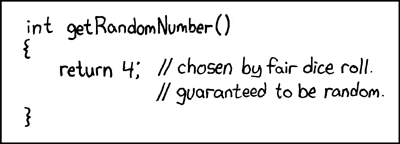## the strange occurrence of the one bump

Posted in Books, Kids, R, Statistics with tags , , , , , , , , on June 8, 2020 by xi'anWhen answering an X validated question on running an accept-reject algorithm for the Gamma distribution by using a mixture of Beta and drifted (bt 1) Exponential distributions, I came across the above glitch in the fit of my 10⁷ simulated sample to the target, apparently displaying a wrong proportion of simulations above (or below) one.

```a=.9
g<-function(T){
x=rexp(T)
v=rt(T,1)<0
x=c(1+x[v],exp(-x/a)[!v])
x[runif(T)<x^a/x/exp(x)/((x>1)*exp(1-x)+a*(x<1)*x^a/x)*a]}```

It took me a while to spot the issue, namely that the output of

```  z=g(T)
while(sum(!!z)<T)z=c(z,g(T))
z[1:T]
```

was favouring simulations from the drifted exponential by truncating. Permuting the elements of z before returning solved the issue (as shown below for a=½)!## CRAN does not validate R packages!

Posted in pictures, R, University life with tags , , , , , , , , , , on July 10, 2019 by xi'anA friend called me the other day for advice on how to submit an R package to CRAN along with a proof his method was mathematically sound. I replied with some items of advice taken from my (limited) experience with submitting packages. And with the remark that CRAN would not validate the mathematical contents of the associated package manual. Nor even the validity of the R code towards delivering the right outcome as stated in the manual. This shocked him quite seriously as he thought having a package accepted by CRAN was a stamp of validation of both the method and the R code. It would be nice of course but would require so much manpower that it seems unrealistic. Some middle ground is to aim at a journal or a peer community validation where both code and methods are vetted. Which happens for instance with the Journal of Computational and Graphical Statistics. Or the Journal of Statistical Software (which should revise its instructions to authors that states “The majority of software published in JSS is written in S, MATLAB, SAS/IML, C++, or Java”. S, really?!)As for the validity of the latest release of R (currently R-3.6.1 which came out on 2019-07-05, named Action of the Toes!), I figure the bazillion R programs currently running should be able to detect any defect pretty fast, although awareness of the incredible failure of sample() reported in an earlier post took a while to appear.

## biased sample!

Posted in Statistics with tags , , , , , , , , , , , on May 21, 2019 by xi'anA chance occurrence led me to this thread on R-devel about R sample function generating a bias by taking the integer part of the continuous uniform generator… And then to the note by Kellie Ottoboni and Philip Stark analysing the reason, namely the fact that R uniform [0,1) pseudo-random generator is not perfectly continuously uniform but discrete, by the nature of numbers on a computer. Knuth (1997) showed that in this case the range of probabilities is larger than (1,1), the largest range being (1,1.03). As noted in the note, exploiting directly the pseudo-random bits of the pseudo-random generator. Shocking, isn’t it!  A fast and bias-free alternative suggested by Lemire is available as `dqsample::sample`

As an update of June 2019, sample is now fixed.

## a perfectly normally distributed sample

Posted in R, Statistics with tags , , , , , , , , on May 9, 2019 by xi'an

When I saw this title on R-bloggers, I was wondering how “more perfect” a Normal sample could be when compared with the outcome of rnorm(n). Hence went checking the original blog on `bayestestR` in search of more information. Which was stating nothing more than how to generate a sample is perfectly normal by using the `rnorm_perfect` function. Still unsure of the meaning, I contacted one of the contributors who replied very quickly

…that’s actually a good question. I would say an empirical sample having characteristics as close as possible to a cannonic gaussian distribution.
and again leaving me hungering for more details. I thus downloaded the package `bayestestR` and opened the `rnorm_perfect` function. Which is simply the sequence of n-quantiles
stats::qnorm(seq(1/n, 1 – 1/n, length.out = n), mean, sd)
which I would definitely not call a sample as it has nothing random. And perfect?! Not really, unless one associates randomness and imperfection.

## Le Monde puzzle [#1001]

Posted in Kids, R with tags , , , , on March 27, 2017 by xi'anAfter a long lag (due to my missing the free copies distributed at Paris-Dauphine!), here is a Sudoku-like Le Monde mathematical puzzle:

A grid of size (n,n) holds integer values such that any entry larger than 1 is the sum of one term in the same column and one term in the same row. What is the maximal possible value observed in such a grid when n=3,4?

This can be solved in R by a random exploration of such possible grids in a simulated annealing spirit:

```mat=matrix(1,N,N)
goal=1

targ=function(mat){ #check constraints
d=0
for (i in (1:(N*N))[mat>1]){
r=(i-1)%%N+1;c=(i-1)%/%N+1
d=d+(min(abs(mat[i]-outer(mat[-r,c],mat[r,-c],"+")))>0)}
return(d)}

cur=0
for (t in 1:1e6){
i=sample(1:(N*N),1);prop=mat
prop[i]=sample(1:(2*goal),1)
d=targ(prop)
if (10*log(runif(1))/t<cur-d){
mat=prop;cur=d}
if ((d==0)&(max(prop)>goal)){
goal=max(prop);maxx=prop}}```

returning a value of 8 for n=3 and 37 for n=4. However, the method is quite myopic and I tried instead a random filling of the grid, using each time the maximum possible sum for empty cells:

```goal=1
for (v in 1:1e6){
mat=matrix(0,N,N)
#one 1 per row/col
for (i in 1:N) mat[i,sample(1:N,1)]=1
for (i in 1:N) if (max(mat[,i])==0) mat[sample(1:N,1),i]=1
while (min(mat)==0){
parm=sample(1:(N*N)) #random order
for (i in parm[mat[parm]==0]){
r=(i-1)%%N+1;c=(i-1)%/%N+1
if ((max(mat[-r,c])>0)&(max(mat[r,-c])>0)){
mat[i]=max(mat[-r,c])+max(mat[r,-c])
break()}}}
if (goal<max(mat)){
goal=max(mat);maxx=mat}}
```

which recovered a maximum of 8 for n=3, but reached 48 for n=4. And 211 for n=5, 647 for n=6… For instance, here is the solution for n=4:

```[1,]    1    5   11   10
[2,]    2    4    1    5
[3,]   48    2   24    1
[4,]   24    1   22   11
```